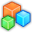# Microsoft Small BasicProgram Listing: KQL710
GraphicsWindow.Width = 400
GraphicsWindow.Height = 300

'Data table
draw = "X=130;Y=80;W=50;H=50;color=blue;tag=eye" 'left
draw = "X=220;Y=80;W=50;H=50;color=green;tag=eye" 'right
draw = "X=180;Y=110;W=40;H=60;color=black;tag=nose"
draw = "X=60;Y=90;W=40;H=60;color=cyan;tag=leftEar"
draw = "X=300;Y=90;W=40;H=60;color=gray;tag=rightEar"
draw = "X=180;Y=180;W=40;H=20;color=purple;tag=mouth"

'Draw outline
For i = 1 To Array.GetItemCount(draw)
GraphicsWindow.PenColor = draw[i]["color"]
GraphicsWindow.DrawEllipse(draw[i]["X"], draw[i]["Y"], draw[i]["W"], draw[i]["H"])
Program.Delay(300)
EndFor

'Add and Animate shapes for solid color
GraphicsWindow.PenWidth = 0
For i = 1 To Array.GetItemCount(draw)
GraphicsWindow.BrushColor = draw[i]["color"]
Shapes.Animate(shp[i], draw[i]["X"], draw[i]["Y"], 500)
Program.Delay(500)
EndFor

'Animate shapes - eyes and mouth
dZ = 0.1
zoom = 1
While 0=0
zoom = zoom - dZ
For i = 1 To Array.GetItemCount(draw)
If Array.ContainsValue(draw[i] "eye") Then
Shapes.Zoom(shp[i] 1 zoom)
ElseIf Array.ContainsValue(draw[i] "mouth") Then
Shapes.Zoom(shp[i] zoom zoom)
EndIf
EndFor
If zoom = 0 Or zoom = 1 Then
dZ = -dZ
EndIf
Program.Delay(100)
EndWhile